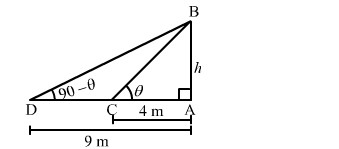# The angles of elevation of the top of a tower from two points at distances of 4 m`
Question:

The angles of elevation of the top of a tower from two points at distances of 4 m and 9 m from the base of the tower and in the same straight line with it are complementary. Show that the height of the tower is 6 metres.

Solution:

Let $A B$ be the tower and $C$ and $D$ be two points such that $A C=4 \mathrm{~m}$ and $A D=9 \mathrm{~m}$.

Let:

$A B=h \mathrm{~m}, \angle B C A=\theta$ and $\angle B D A=90^{\circ}-\theta$In the right $\triangle B C A$, we have:

$\tan \theta=\frac{A B}{A C}$

$\Rightarrow \tan \theta=\frac{h}{4} \quad \ldots(1)$

In the right ∆BDA, we have:tan90°-θ=ABADcot θ=h9                  tan90°-θ=cot θ1tan θ=h9       ...(2)     cot θ=1tan θ">

$\tan \left(90^{\circ}-\theta\right)=\frac{A B}{A D}$

$\Rightarrow \cot \theta=\frac{h}{9} \quad\left[\tan \left(90^{\circ}-\theta\right)=\cot \theta\right]$

$\Rightarrow \frac{1}{\tan \theta}=\frac{h}{9} \quad \ldots(2) \quad\left[\cot \theta=\frac{1}{\tan \theta}\right]$

Multiplying equations (1) and (2), we get:

$\tan \theta \times \frac{1}{\tan \theta}=\frac{h}{4} \times \frac{h}{9}$

$\Rightarrow 1=\frac{h^{2}}{36}$

$\Rightarrow 36=h^{2}$

⇒ h = ±6

Height of a tower cannot be negative.
∴ Height of the tower = 6 m